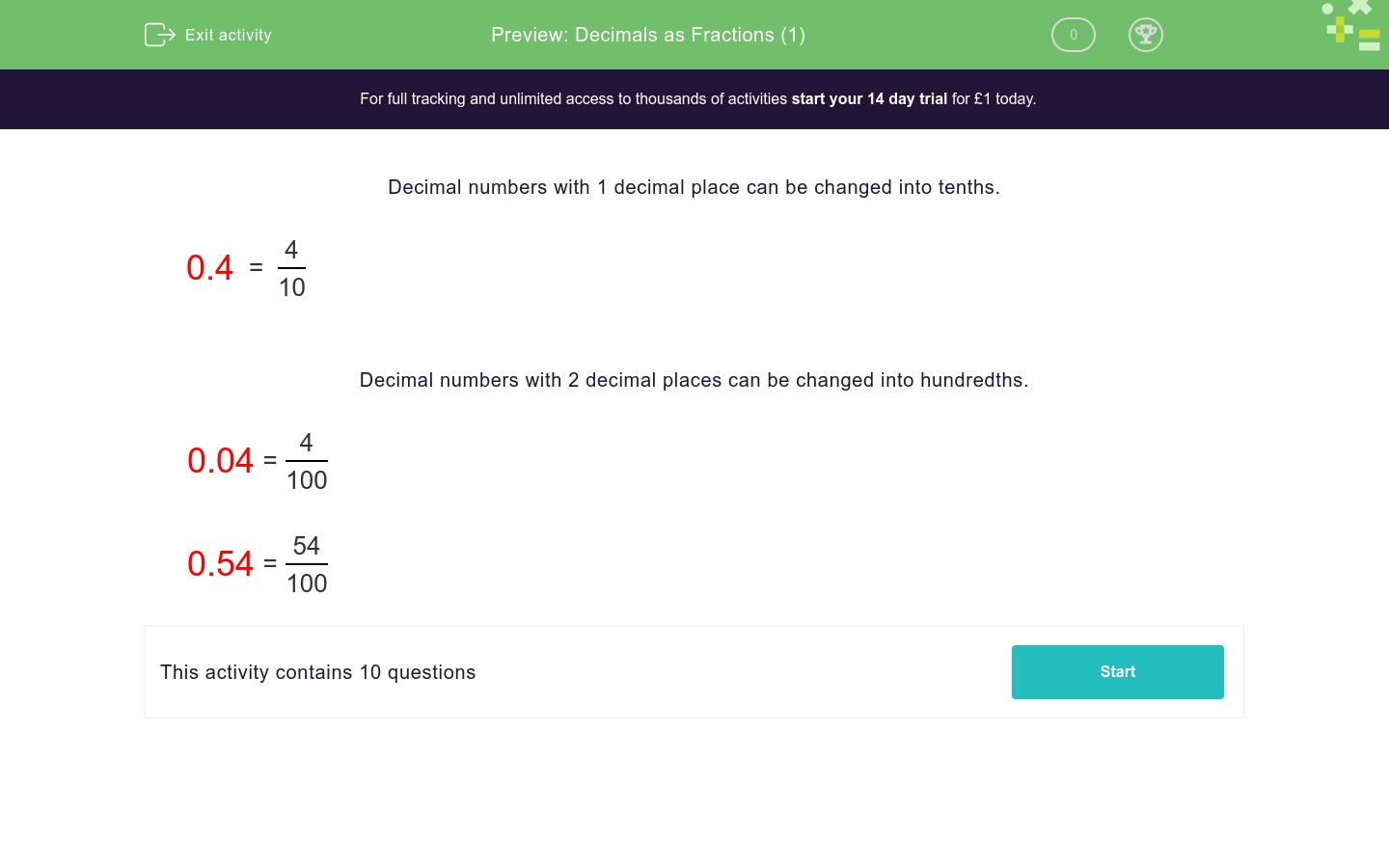# Decimals as Fractions (1)

In this worksheet, students must read and write decimal numbers as fractions.Key stage:  KS 2

Curriculum topic:  Number: Fractions, Decimals and Percentages

Curriculum subtopic:  Know Decimal Numbers

Difficulty level:### QUESTION 1 of 10

Decimal numbers with 1 decimal place can be changed into tenths.

 0.4 = 4 10

Decimal numbers with 2 decimal places can be changed into hundredths.

 0.04 = 4 100

 0.54 = 54 100

Change this decimal to a fraction.

Choose the right answer.

0.8

8/10

8/100

10/8

Change this decimal to a fraction.

Choose the right answer.

0.2

2/10

2/100

10/2

Change this decimal to a fraction.

Choose the right answer.

0.12

12/10

12/100

10/12

Change this decimal to a fraction.

Choose the right answer.

0.7

7/10

7/100

7/1000

Change this decimal to a fraction.

Choose the right answer.

0.07

7/10

7/100

7/1000

Change this decimal to a fraction.

Choose the right answer.

0.01

1/10

1/100

1/1000

Change this decimal to a fraction.

Choose the right answer.

0.61

61/10

61/100

61/1000

Change this decimal to a fraction.

Choose the right answer.

0.09

9/10

9/100

10/9

Change this decimal to a fraction.

Choose the right answer.

0.61

61/10

61/100

61/1000

Change this decimal to a fraction.

Choose the right answer.

0.99

99/10

99/1000

99/100

• Question 1

Change this decimal to a fraction.

Choose the right answer.

0.8

8/10
EDDIE SAYS
1 decimal place so 8 tenths.
• Question 2

Change this decimal to a fraction.

Choose the right answer.

0.2

2/10
EDDIE SAYS
1 decimal place so 2 tenths.
• Question 3

Change this decimal to a fraction.

Choose the right answer.

0.12

12/100
EDDIE SAYS
2 decimal places so 12 hundredths.
• Question 4

Change this decimal to a fraction.

Choose the right answer.

0.7

7/10
EDDIE SAYS
1 decimal place so 7 tenths.
• Question 5

Change this decimal to a fraction.

Choose the right answer.

0.07

7/100
EDDIE SAYS
2 decimal places so 7 hundredths.
• Question 6

Change this decimal to a fraction.

Choose the right answer.

0.01

1/100
EDDIE SAYS
2 decimal places so 1 hundredth.
• Question 7

Change this decimal to a fraction.

Choose the right answer.

0.61

61/100
EDDIE SAYS
2 decimal places so 61 hundredths.
• Question 8

Change this decimal to a fraction.

Choose the right answer.

0.09

9/100
EDDIE SAYS
2 decimal places so 9 hundredths.
• Question 9

Change this decimal to a fraction.

Choose the right answer.

0.61

61/100
EDDIE SAYS
2 decimal places so 61 hundredths.
• Question 10

Change this decimal to a fraction.

Choose the right answer.

0.99

99/100
EDDIE SAYS
2 decimal places so 99 hundredths.
---- OR ----

Sign up for a £1 trial so you can track and measure your child's progress on this activity.

### What is EdPlace?

We're your National Curriculum aligned online education content provider helping each child succeed in English, maths and science from year 1 to GCSE. With an EdPlace account you’ll be able to track and measure progress, helping each child achieve their best. We build confidence and attainment by personalising each child’s learning at a level that suits them.

Get startedStart your £1 trial today.
Subscribe from £10/month.